# Why error message #DIV0! occurs and how to solve it

Uploaded time: February 24, 2022 Difficulty Intermediate

A free Office suite fully compatible with Microsoft OfficeWhy error message #DIV0! occurs and how to solve it

# Why error message #DIV0! occurs and how to solve it

When calculating data with WPS Spreadsheet, the error message #DIV/0! will come to us from time to time.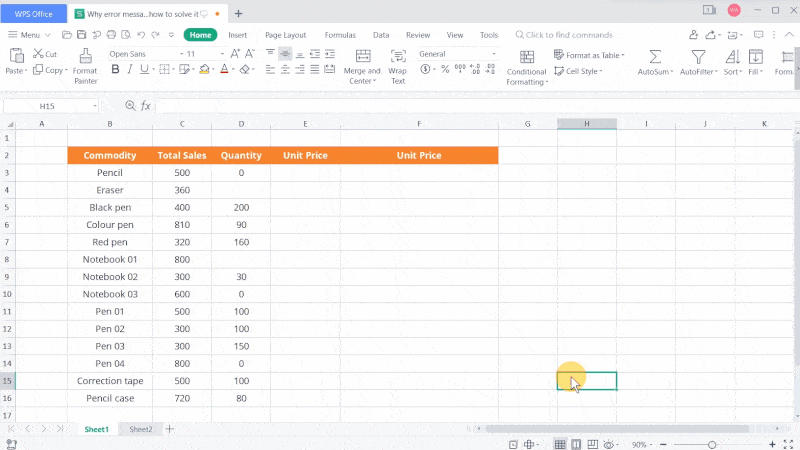What caused the mistake? And how can we remove it?

Take this table as an example. For example, when we want to calculate the unit price of pencils, we can enter =C3/D3 in cell E3, and the calculation result will show the error value #DIV/0!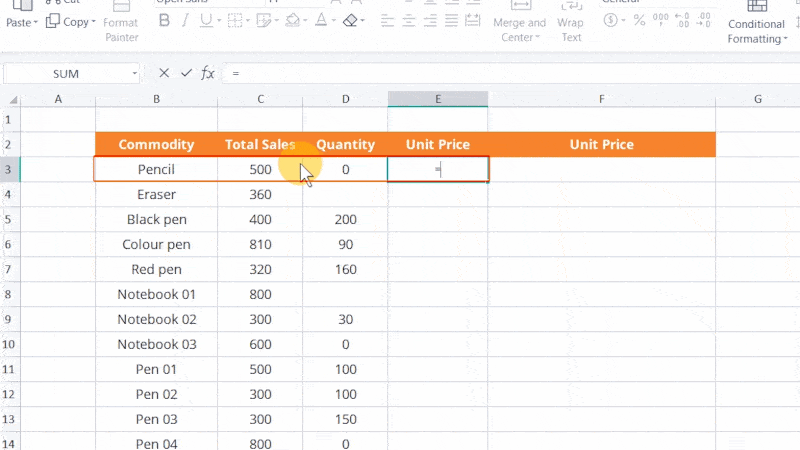This is because, in this division operation, the denominator is 0, which leads to the calculation error.

When we drop down and fill in the calculation result, we will find that the error value also occurs when the denominator is a blank.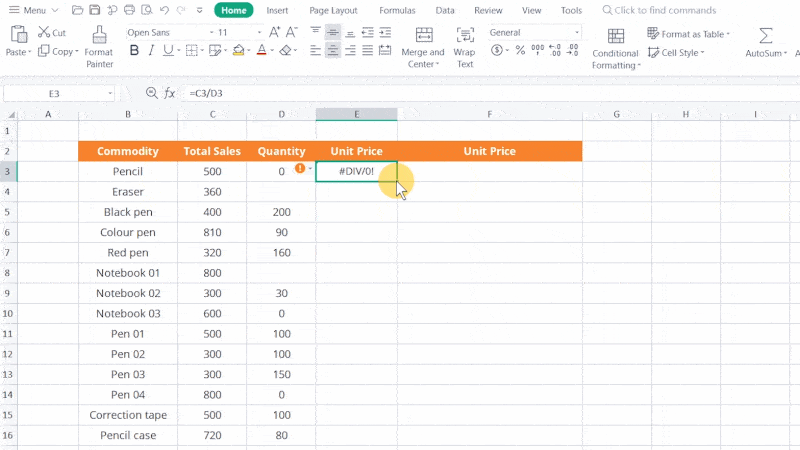If the table contains mass data, we can use the IF function to judge. For example, enter the following formula in cell F3:

= IF(D3=0, Denominator cannot be 0, C3/D3).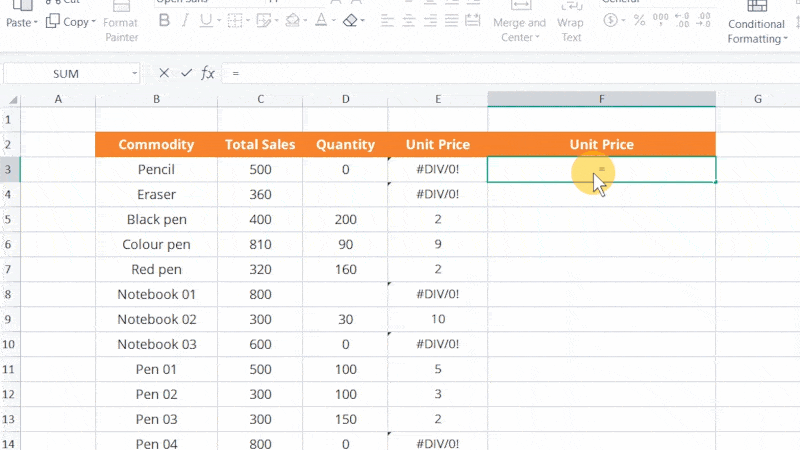The IF function will determine whether the value in D3 cell is 0. If TRUE, it will return The denominator cannot be 0. If FALSE, it will return the result of C3 divided by D3.

Note: Double quotation marks should be added to text.

Of course, we can also customize the return value.

In addition, the error value #DIV/0! might occur when calculating the average value.

Take this score sheet as an example. We want to find out the average grade of students whose physics scores are lower than 60 and enter the following formula in cell H2 =AVERAGEIF(F2:F15,.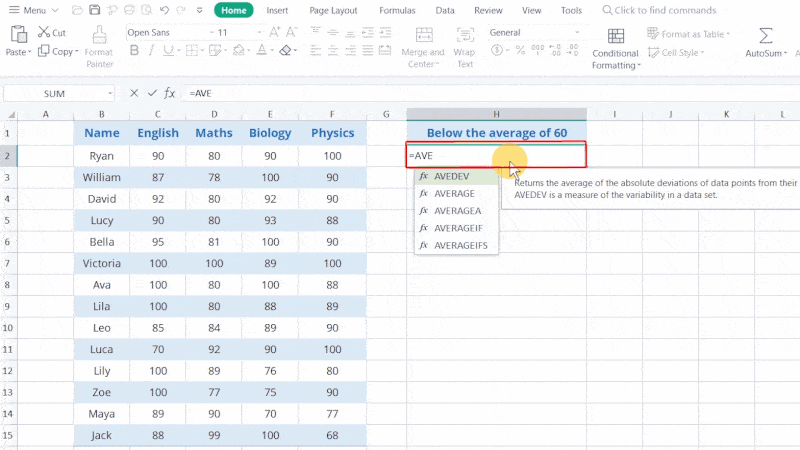This means that the AVERAGEIF function is used to calculate whether the average value of cells in F2:F15 meets the condition of

Click Enter, then you can see that #DIV/0! appears in cell H2, because there are no cells in F2:F15 that meets the condition of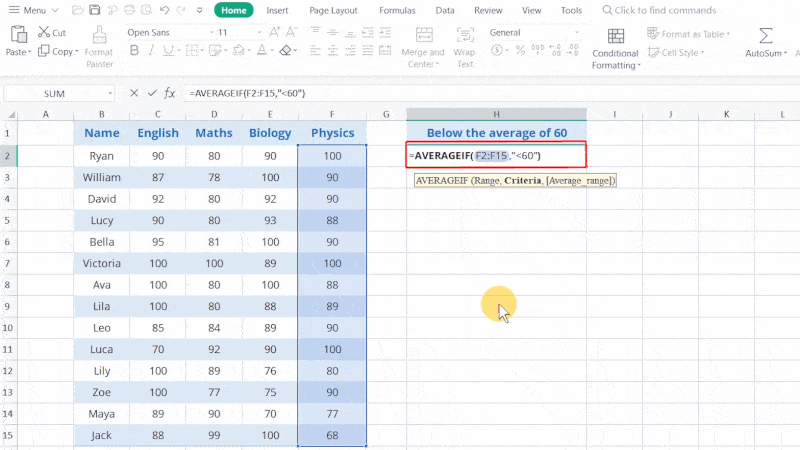Therefore, if the divisor is 0, the error value #DIV/0! will appear .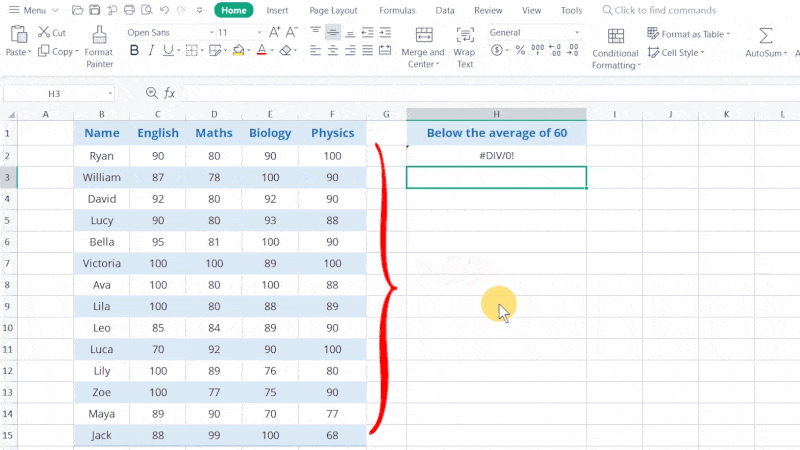We can avoid such mistakes through today's tutorial.

WPS Office Spreadsheet is helpful for various fields of studies: mathematics, statistics, data science, engineering, finance, and such. Rich in functions paired with teaching procedures, WPS Office Spreadsheet definitely is a professional and reliable toolkit.